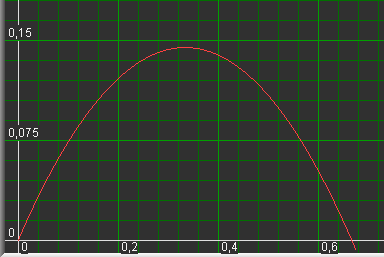the Ultimate GutterLuctor et Emergo(Pump or Drawn)
From the Netherlands, land reclaimed from sea, with it's canals, locks, dikes, ditches
and windmills, a search for the Ultimate Gutter Dimensions..

See section below:In this trapezium the total length AB + BC + CD = 1.

Variables are angle a and length x.

Question: for which values of a and x, will area ABCD have a maximum?

Two solutions are presented:Dutch windmill
A formula for the area ABCD
See picture above::
The area A is the addition of a rectangle (1-2x)h
and two triangles, together bh, so:
A = (1 - 2x)h + bh
Note:
h = x.sin(a)
b = x.cos(a)
so
A = x2.sin(a).cos(a) + (1 - 2x).x.sin(a)
A = x2.sin(a).cos(a) + x.sin(a) - 2x2.sin(a)

the Graphical solution
Graphics-Explorer uses variables x en y.
Also, constants (a,b,c) may be used in formula's.
These constants are changeble by mouseclick, graphics adjust to new values.
It's obvious to use x for a side, y for the area and a for the angle a.

Type the formula:
y = x^2*sin(a)*cos(a) + x*sin(a) - 2x^2*sin(a)
and change the following settings of Graphics-Explorer:
- coordinates (0,0) left-bottom
- +/- value for constants increment: 5 (degrees)
- zoom-center at (0,0)
- x- scale (1) at right side (x < 1)
- y- scale (0.2) at top
- "Autoplot" (to make graphics adjust on constants change)
- "replace" (to enable cleanup of old graphics)
Plot the formula and change value of a.
Observe maximum value of y and read values of x en a, see picure below:While plotting the formula with different values of a, we notice that y has a maximum when the angle
is near 60 degrees and bottom and sides have about equal lengths.

The analytical approach
The maximum or minimum of a function can be found by differentiation.
The derivative of a function equals 0 in this case.
There are 2 variables, x and a,
so, for a maximum of y both the derivatives of A to x as well as A to a must be 0.

Differentiation of A to x
 d A d x
= 2 x ·  sin a ·  cos a +  sin a − 4 x ·  sin a = 0

2 x ·  cos a + 1 − 4 x = 0
2 x ·  cos a = 4 x − 1
cos a =  4 x − 1 2 x
Differentiation of A to a
 d A d a
= x 2 (( cos a) 2 − ( sin a) 2) + x ·  cos a − 2 x 2 ·  cos a = 0

x 2 ( cos a) 2 − x 2 ( sin a) 2 + x ·  cos a − 2 x 2 ·  cos a = 0
x ( cos a) 2 − x ( sin a) 2 +  cos a − 2 x ·  cos a = 0
x ( cos a) 2 − x + x ( cos a) 2 +  cos a − 2 x ·  cos a = 0
2 x ( cos a) 2 + (1 − 2 x) ·  cos a − x = 0
Now substitute cos(a):
2 x
2
æ 4 x − 1 2 x
ö
­­
èø
+  (1 − 2 x) (4 x − 1) 2 x
− x = 0

(4 x − 1) 2 + (1 − 2 x) (4 x − 1) − 2 x 2 = 0
16 x 2 − 8 x + 1 + 4 x − 1 − 8 x 2 + 2 x − 2 x 2 = 0
6 x 2 − 2 x = 0
x =  1 3
Finally, use x to find the best value of a:

cos a

=

 4 3
− 1
 2 3

=

 1 2

So: a = 60 degrees.

The ultimate gutter has sides with an angle of 60 degrees. Bottom and sides have equal lengths.